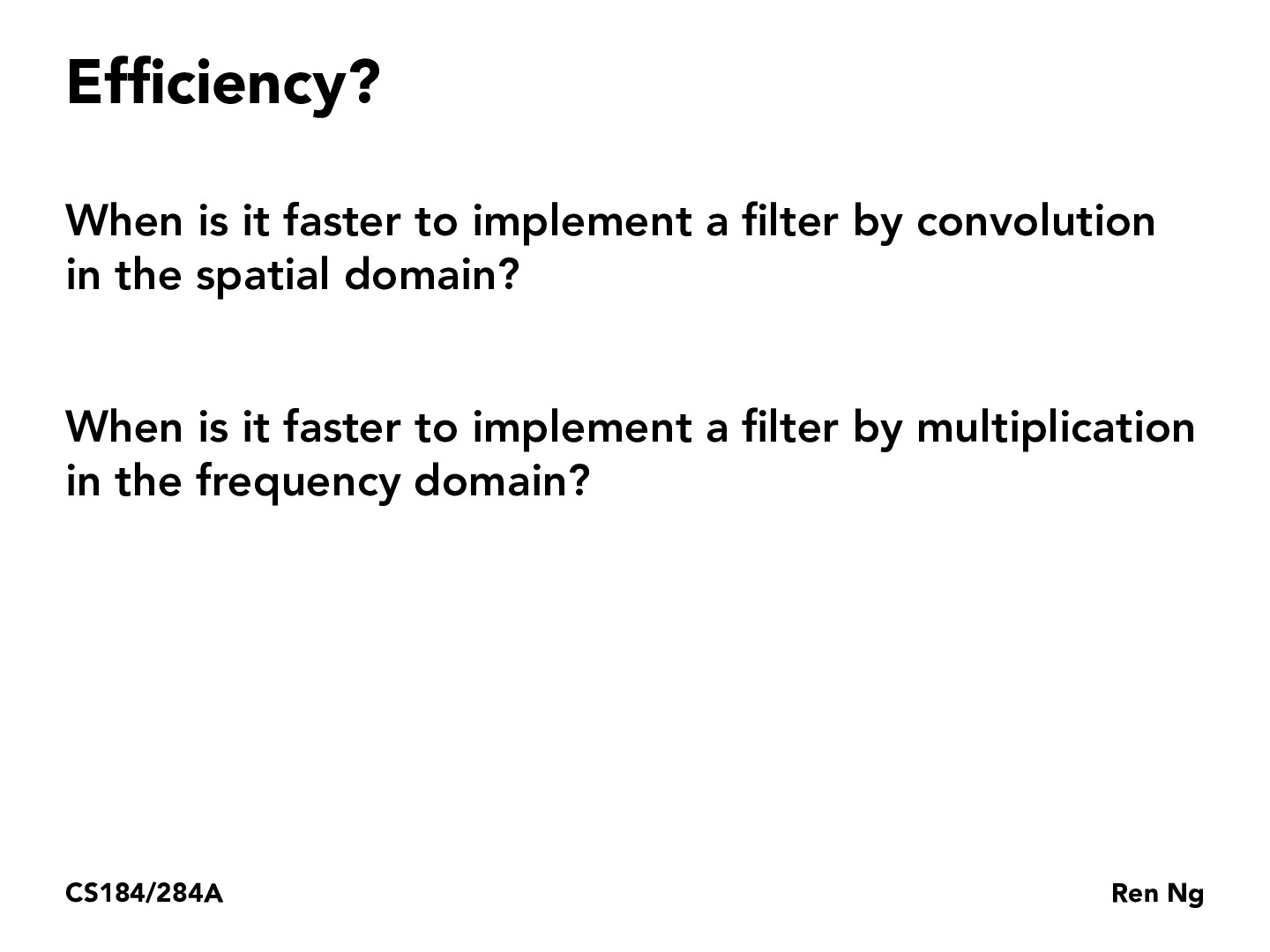You are viewing the course site for a past offering of this course. The current offering may be found here.Lecture 3: Sampling, Aliasing, Antialiasing (60)JiaweiChenKodomo

Here is my thinking. Suppose the image has $n$ pixels and the filter has $m$ pixels in total. Doing convolution in spatial domain will cost $\mathcal{O}(nm)$, as for each pixel there are $m$ multiplications and summations.

Doing Fast Fourier Transform (and the reverse transform?) of the image costs $\mathcal{O}(n\log(n))$, and doing multiplication costs $\mathcal{O}(n)$ as we are doing it by "pixel" (frequency). The cost of transforming the filter consts $\mathcal{O}(m\log(m))$. Then in total the cost is $\mathcal{O}(n\log(n) + n + m\log(m))$, or $\mathcal{O}(n\log(n))$ if $m$ is ignorable against $n$.

Then we choose the most efficient one.

What do you guys think?liboan

I generally agree with this.

I am curious tho if you can take advantage of parallelism/data locality to speed up the convolution. Because depending on the stride length of the convolution, you may be able to apply several convolutions to the image at once.katamarisun

Great analysis @Jiawei! @liboan gives a good response, and it is important to note that while 20-30 years ago FFT would certainly be the faster computation, with the rise of GPUs parallelism, and convolution -> matrix unrolling for CNN processors, CNNs on the raw matrix is often faster, especially considering the small size of most convolution matrices. Asymptotically, FFT would be better, but at small dimensions the constants are very important factors.

You must be enrolled in the course to comment